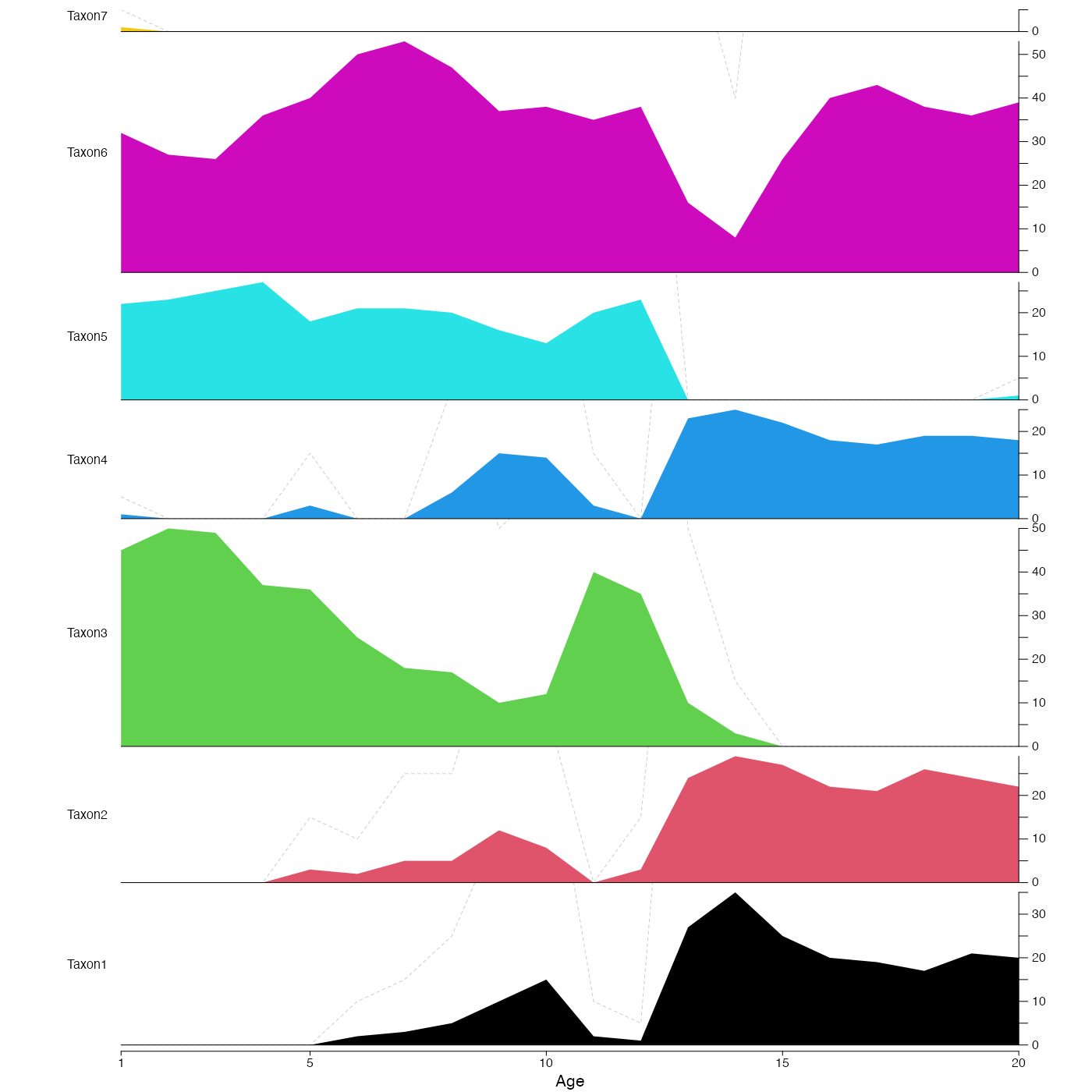This function plots stratigraphic data either as polygons or bars.

plot_diagram(
x,
bars = FALSE,
col = "black",
amplif = 5,
save = FALSE,
filename = "Diagram.pdf",
width = 3.54,
height = 9,
as.png = FALSE,
png.res = 300,
yax_incr = 5,
bar_width = diff(range(x$inputs$x))/50,
xlim = NA,
tickAtSample = TRUE,
col_pos = "black",
col_neg = "grey80",
title = NA,
src = NA
)

## Arguments

x

A data frame of the data to plot (first column with age or depth) and the taxa in the following columns. x can also be a crestObj.

bars

A boolean that indicates if the data should be plotted as polygons (default: bars=FALSE) or vertical bars (bars=TRUE).

col

Colours to be used for the polygons. If the number of colours does not match the number of taxa, colors will be recycled.

amplif

A factor the show exaggeration on the diagram. Only for polygon plot. Default 5.

save

A boolean to indicate if the diagram should be saved as a pdf file. Default is FALSE.

filename

An absolute or relative path that indicates where the diagram should be saved. Also used to specify the name of the file. Default: the file is saved in the working directory under the name 'Diagram.pdf'.

width

The width of the output file in inches (default 3.54in ~ 9cm).

height

The height of the output file in inches (default 9in ~ 23cm).

as.png

A boolean to indicate if the output should be saved as a png. Default is FALSE and the figure is saved as a pdf file.

png.res

The resolution of the png file (default 300 pixels per inch).

yax_incr

Graphical parameters describing the increment size on the y-axis (default 5).

bar_width

Width of the bars of the barplot (default 1/50th of the x range).

xlim

The range covered by the x-axis. Can be adjusted to get round numbers on the x-axis. If smaller than the range covered by the data, the data will be truncated (default: range of the data).

tickAtSample

Boolean that indicates whether a tick mark should be added on the x-axis at the location of each sample (default TRUE).

col_pos

Graphical parameter for the barplot. Colour of all the positive values (default black).

col_neg

Graphical parameter for the barplot. Colour of all the negative values (default light grey).

title

Name to be added on top of the plot (default NA).

src

A graphical parameter used by the plot_loo() function.

## Value

No return value, this function is used to plot.

## Examples

data(crest_ex)
plot_diagram(crest_ex, bars=TRUE, col='black', bar_width=0.8)plot_diagram(crest_ex,  col=1:7, tickAtSample=FALSE)#> Replace 'tempdir()' by the location where the sample should be saved (e.g. 'getwd()')
plot_diagram(crest_ex, save=TRUE,
filename=file.path(tempdir(), 'testDiagram.pdf'),
bars=TRUE, col_pos='cornflowerblue', col_neg='darkgreen',
bar_width=0.8, xlim=c(3,15))